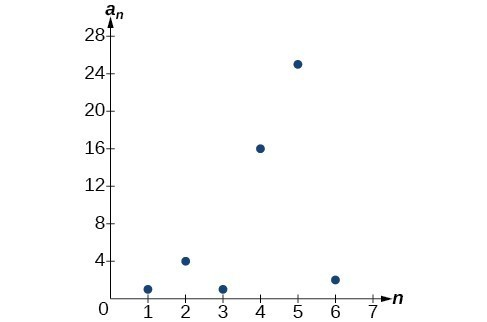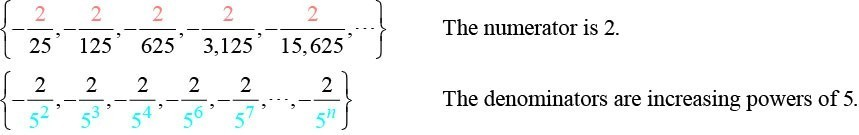## Investigating Explicit Formulas

We’ve learned that sequences are functions whose domain is over the positive integers. This is true for other types of functions, including some piecewise functions. Recall that a piecewise function is a function defined by multiple subsections. A different formula might represent each individual subsection.

### How To: Given an explicit formula for a piecewise function, write the first $n$ terms of a sequence

1. Identify the formula to which $n=1$ applies.
2. To find the first term, ${a}_{1}$, use $n=1$ in the appropriate formula.
3. Identify the formula to which $n=2$ applies.
4. To find the second term, ${a}_{2}$, use $n=2$ in the appropriate formula.
5. Continue in the same manner until you have identified all $n$ terms.

### Example 3: Writing the Terms of a Sequence Defined by a Piecewise Explicit Formula

Write the first six terms of the sequence.

${a_{n}}=\begin{cases}n^{2} \hfill& \text{if }n\text{ is not divisible by 3} \\ \frac{n}{3} \hfill& \text{if }n\text{ is divisible by 3}\end{cases}$

### Solution

Substitute $n=1,n=2$, and so on in the appropriate formula. Use ${n}^{2}$ when $n$ is not a multiple of 3. Use $\frac{n}{3}$ when $n$ is a multiple of 3.

$\begin{array}{ll}{a}_{1}={1}^{2}=1\begin{array}{cccc}& & & \end{array}\hfill & \text{1 is not a multiple of 3}\text{. Use }{n}^{2}.\hfill \\ {a}_{2}={2}^{2}=4\hfill & \text{2 is not a multiple of 3}\text{. Use }{n}^{2}.\hfill \\ {a}_{3}=\frac{3}{3}=1\hfill & \text{3 is a multiple of 3}\text{. Use }\frac{n}{3}.\hfill \\ {a}_{4}={4}^{2}=16\hfill & \text{4 is not a multiple of 3}\text{. Use }{n}^{2}.\hfill \\ {a}_{5}={5}^{2}=25\hfill & \text{5 is not a multiple of 3}\text{. Use }{n}^{2}.\hfill \\ {a}_{6}=\frac{6}{3}=2\hfill & \text{6 is a multiple of 3}\text{. Use }\frac{n}{3}.\hfill \end{array}$

The first six terms are $\left\{1,\text{ }4,\text{ }1,\text{ }16,\text{ }25,\text{ }2\right\}$.

### Analysis of the Solution

Every third point on the graph shown in Figure 5 stands out from the two nearby points. This occurs because the sequence was defined by a piecewise function.Figure 5

### Try It 3

Write the first six terms of the sequence.

${a_{n}}=\begin{cases}2n^{3} \hfill& \text{if }n\text{ is odd} \\ \frac{5n}{2} \hfill& \text{if }n\text{ is even}\end{cases}$

Solution

## Finding an Explicit Formula

Thus far, we have been given the explicit formula and asked to find a number of terms of the sequence. Sometimes, the explicit formula for the $n\text{th}$ term of a sequence is not given. Instead, we are given several terms from the sequence. When this happens, we can work in reverse to find an explicit formula from the first few terms of a sequence. The key to finding an explicit formula is to look for a pattern in the terms. Keep in mind that the pattern may involve alternating terms, formulas for numerators, formulas for denominators, exponents, or bases.

### How To: Given the first few terms of a sequence, find an explicit formula for the sequence.

1. Look for a pattern among the terms.
2. If the terms are fractions, look for a separate pattern among the numerators and denominators.
3. Look for a pattern among the signs of the terms.
4. Write a formula for ${a}_{n}$ in terms of $n$. Test your formula for $n=1,\text{ }n=2$, and $n=3$.

### Example 4: Writing an Explicit Formula for the nth Term of a Sequence

Write an explicit formula for the $n\text{th}$ term of each sequence.

1. $\left\{-\frac{2}{11},\frac{3}{13},-\frac{4}{15},\frac{5}{17},-\frac{6}{19},\dots \right\}$
2. $\left\{-\frac{2}{25}\text{,}-\frac{2}{125}\text{,}-\frac{2}{625}\text{,}-\frac{2}{3\text{,}125}\text{,}-\frac{2}{15\text{,}625}\text{,}\dots \right\}$
3. $\left\{{e}^{4}\text{,}{e}^{5}\text{,}{e}^{6}\text{,}{e}^{7}\text{,}{e}^{8}\text{,}\dots \right\}$

### Solution

Look for the pattern in each sequence.

1. The terms alternate between positive and negative. We can use ${\left(-1\right)}^{n}$ to make the terms alternate. The numerator can be represented by $n+1$. The denominator can be represented by $2n+9$.

${a}_{n}=\frac{{\left(-1\right)}^{n}\left(n+1\right)}{2n+9}$

2. The terms are all negative.So we know that the fraction is negative, the numerator is 2, and the denominator can be represented by ${5}^{n+1}$.

${a}_{n}=-\frac{2}{{5}^{n+1}}$
3. The terms are powers of $e$. For $n=1$, the first term is ${e}^{4}$ so the exponent must be $n+3$.
${a}_{n}={e}^{n+3}$

### Try It 4

Write an explicit formula for the $n\text{th}$ term of the sequence.

$\{9;−81,729;−6,561;59,049\}$

### Try It 5

Write an explicit formula for the $n\text{th}$ term of the sequence.

$\left\{-\frac{3}{4},-\frac{9}{8},-\frac{27}{12},-\frac{81}{16},-\frac{243}{20},…\right\}$

### Try It 6

Write an explicit formula for the $n\text{th}$ term of the sequence.

$\left\{\frac{1}{{e}^{2}}, \frac{1}{e}, 1, e, {e}^{2},…\right\}$

Solution